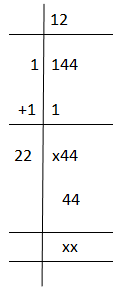Checkout JEE MAINS 2022 Question Paper Analysis : Checkout JEE MAINS 2022 Question Paper Analysis :

# Square Root of 144

The value of the square root of 144 is equal to 12. In radical form, it is denoted as √144 = 12. To calculate the value to root 144 without using a calculator, there are two methods: one is the prime factorisation and another is the long division method. We will learn both the methods here, which will help students to find the root of any number easily and in the fastest way. Also, learn here about square root in a detailed way.

 Square root of 144, √144 = 12

As per the given expression above, we can define the square root of natural number 144, is a value which on multiplying by itself gives 144.

Hence, 12 x 12 = 144 or 122 = 144

## Simplification of Square Root of 144

Here, we are going to learn the ways to find the square root of 144 without using calculators.

Prime Factorisation:

In prime factorisation, we have to find the prime factors in the given number. This method is easy to apply, as we have learned about prime factors in our earlier classes. But, it can only be used if a number is a perfect square.

Clearly, 144 is a perfect square, thus the prime factors are,

144 = 2x2x2x2x3x3

Therefore, if we take the square root on both the sides, we get;

√144 = √(2x2x2x2x3x3)

As we can see here, there are two pairs of 2 and one pair of 3. Hence,

√144 = 2x2x3

√144 = 12

Long Division method

We can also find the square root of any number using long division method. This method is very useful and is the fastest method of all to find the root. It can be used to find the root of imperfect squares and of large numbers, which is not possible in the case of prime factorisation. Below are the steps for using the long division method.

• Take the first digit i.e. 1 and leave the rest two digits i.e. 4
• Now the square of 1 is 1. Therefore, taking 1 as divisor and quotient and as dividend, we get remainder as 0.
• Now, we will take down the other two numbers, i.e. 44, as dividend and add 1 to the divisor to get our next divisor, i.e. 1+1 =2.
• Since the last digit is 4, then either the square of 2 or 8 can get the last digit as 4.
• Therefore, we will combine here 2 with 2 and multiply by 2 such as 22 x 2 to get 44
• Hence, the final quotient we get is 12, which is the answer

Below are the solved steps to take the root of 144.## Video Lessons on Square Roots

### Visualising square roots### Finding Square rootsFind more square roots below:

Test your Knowledge on Square Root of 144